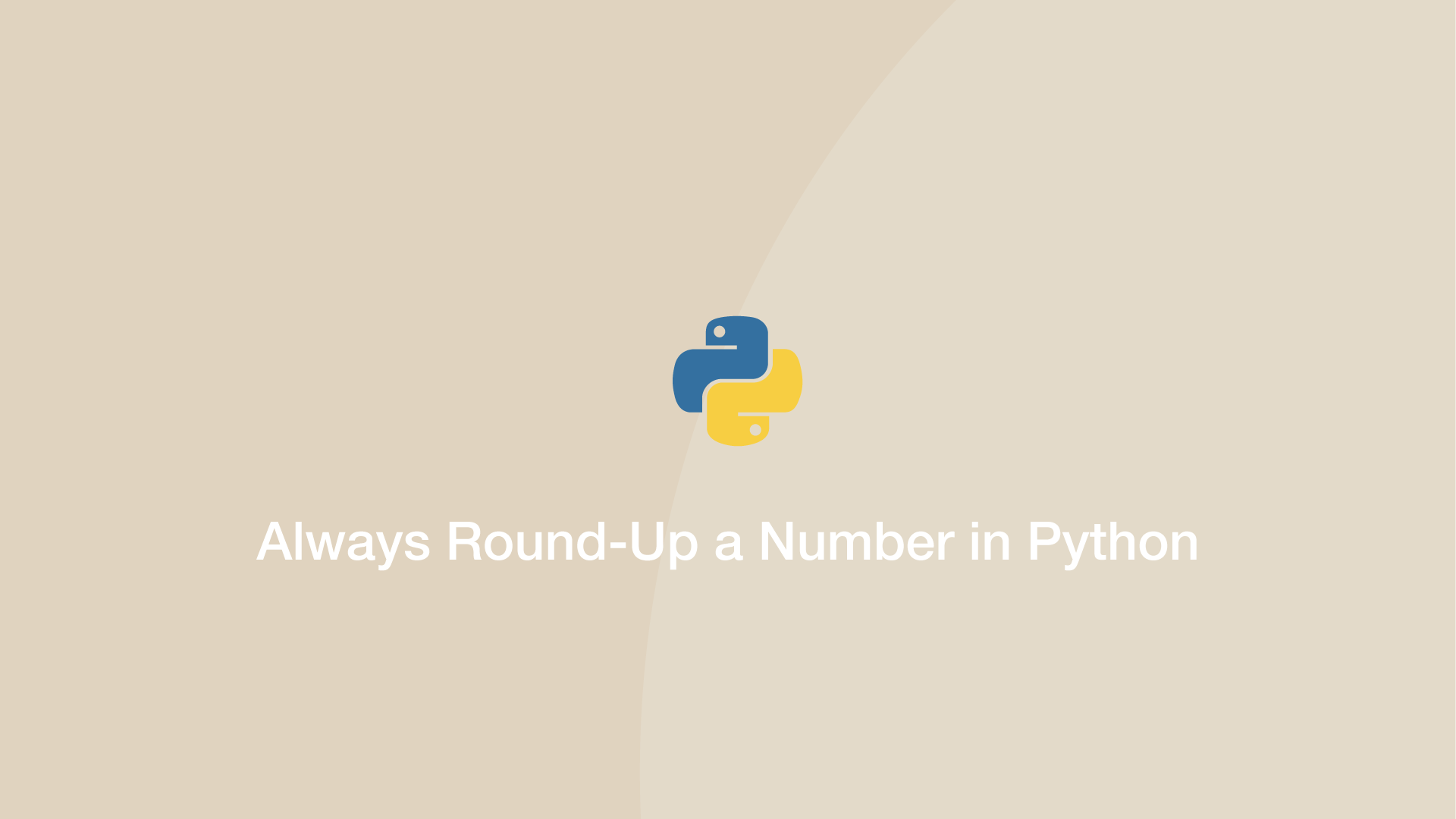# How to Always Round-Up a Number in PythonTo always round up a number regards whether the decimal place to evaluate it closer to 0 than 9 or not use the `ceil()` function from the Python math package.

## Round Number Up with the math.ceil() Function

Let's demonstrate this, let's round up a number that would normally be rounded down.

``````import math
print(int(math.ceil(5.2)))
``````
``````6
``````

## Use the NumPy ceil() Function to Always Round up Numbers

NumPy has a built-in `ceil()` function that you might prefer if you are already using this package. Call it like this:

``````import numpy as np
np.ceil(4.3)
``````
``````5.0
``````

#### Related Tutorials### How to Round Numbers Up and Down in Python

September 28, 2020### How to Round Decimals Up and Down in JavaScript

September 08, 2020### Truncate Float in Python

June 29, 2021### How to Divide in Python

April 01, 2021### How to Create and Use Arrays in JavaScript

July 11, 2020October 07, 2020